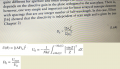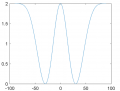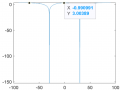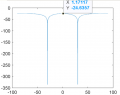# problem implementing directivity of array factor in matlab

#### yef smith

Joined Aug 2, 2020
559
Hello, I am trying to impelemnt in matlab directivity of an array factor .
first i have implemented array factor using formula 1 but instead of cosine i have used sine.
then i have combined two definitions from books as shown bellow and tried to implement them into matlab.
first i have tried to plot simple 2 element array factor as shown bellow and i get the logical 3dB.

but when i have built the formula in matlab exactly as shown by the formulas as shown bellow i get directivity of -24 dB.
where did i go wrong?
Thanks.
Code:
clc
clear all
theta=linspace(-pi/2,pi/2,1000);
theta_0=pi*(0/180);
f=10;

N=2;

lambda=300/f;
k=(2*pi)/lambda;
d=1*lambda;

delta=-k*d*sin(theta_0);
ksi=k*d.*sin(theta)+delta;
AF2=0;
for m=1:N
AF2=AF2+exp(1i*(m-1)*(k*d*sin(theta)+delta));
end

dir=(AF2.^2)./(sum(1+(exp(1i*(k*d*sin(theta)+delta))).^2));
plot(theta/pi*180,10*log10(dir))